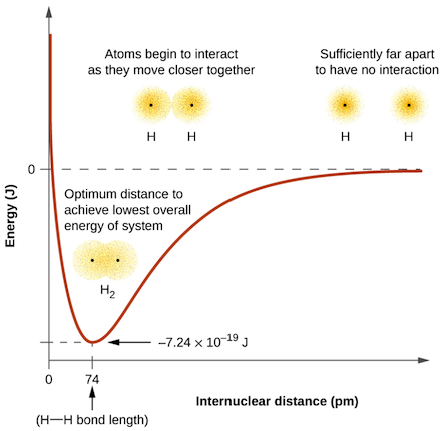# Problem: Explain why bonds occur at specific average bond distances instead of the atoms approaching each other infinitely close.

###### FREE Expert Solution
84% (280 ratings)
###### Problem Details

Explain why bonds occur at specific average bond distances instead of the atoms approaching each other infinitely close.What scientific concept do you need to know in order to solve this problem?

Our tutors have indicated that to solve this problem you will need to apply the Orbital Overlap concept. If you need more Orbital Overlap practice, you can also practice Orbital Overlap practice problems.

What is the difficulty of this problem?

Our tutors rated the difficulty ofExplain why bonds occur at specific average bond distances i...as medium difficulty.

How long does this problem take to solve?

Our expert Chemistry tutor, Dasha took 3 minutes and 51 seconds to solve this problem. You can follow their steps in the video explanation above.

What professor is this problem relevant for?

Based on our data, we think this problem is relevant for Professor Nunez's class at UK.

What textbook is this problem found in?

Our data indicates that this problem or a close variation was asked in Chemistry - OpenStax 2015th Edition. You can also practice Chemistry - OpenStax 2015th Edition practice problems.